Ch.3 - Chemical ReactionsWorksheetSee all chapters
 Ch.1 - Intro to General Chemistry 2hrs & 53mins 0% complete Worksheet Ch.2 - Atoms & Elements 2hrs & 40mins 0% complete Worksheet Ch.3 - Chemical Reactions 3hrs & 25mins 0% complete Worksheet BONUS: Lab Techniques and Procedures 1hr & 38mins 0% complete Worksheet BONUS: Mathematical Operations and Functions 47mins 0% complete Worksheet Ch.4 - Chemical Quantities & Aqueous Reactions 3hrs & 30mins 0% complete Worksheet Ch.5 - Gases 3hrs & 47mins 0% complete Worksheet Ch.6 - Thermochemistry 2hrs & 28mins 0% complete Worksheet Ch.7 - Quantum Mechanics 2hrs & 35mins 0% complete Worksheet Ch.8 - Periodic Properties of the Elements 1hr & 57mins 0% complete Worksheet Ch.9 - Bonding & Molecular Structure 2hrs & 5mins 0% complete Worksheet Ch.10 - Molecular Shapes & Valence Bond Theory 1hr & 31mins 0% complete Worksheet Ch.11 - Liquids, Solids & Intermolecular Forces 3hrs & 40mins 0% complete Worksheet Ch.12 - Solutions 2hrs & 17mins 0% complete Worksheet Ch.13 - Chemical Kinetics 2hrs & 22mins 0% complete Worksheet Ch.14 - Chemical Equilibrium 2hrs & 26mins 0% complete Worksheet Ch.15 - Acid and Base Equilibrium 4hrs & 42mins 0% complete Worksheet Ch.16 - Aqueous Equilibrium 3hrs & 48mins 0% complete Worksheet Ch. 17 - Chemical Thermodynamics 1hr & 44mins 0% complete Worksheet Ch.18 - Electrochemistry 2hrs & 58mins 0% complete Worksheet Ch.19 - Nuclear Chemistry 1hr & 33mins 0% complete Worksheet Ch.20 - Organic Chemistry 3hrs 0% complete Worksheet Ch.22 - Chemistry of the Nonmetals 2hrs & 1min 0% complete Worksheet Ch.23 - Transition Metals and Coordination Compounds 1hr & 54mins 0% complete Worksheet

# Molecular Formula

See all sectionsJules Bruno

The molecular formula represents the true ratio of atoms, whose subscripts show the actual number of each type of atom, in a given chemical compound. It is closely linked to the molar mass or molecular mass of a chemical compound.

Molecular Formula vs. Empirical Formula

Determining the molecular formula of a chemical compound usually first involves finding its empirical formula, which represents the simple or condensed formula.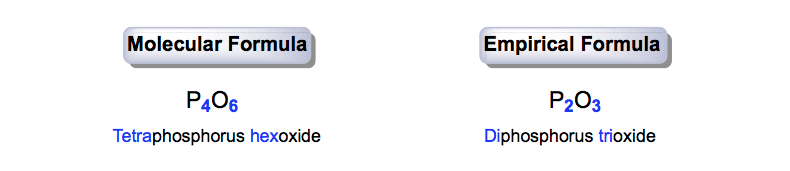Molecular Formula vs. Empirical Formula

Tetraphosphorus hexoxide (P4O6) represents the true formula for the chemical compound. Now since both 4 and 6 are divisible by 2 we can reduce the molecular formula to diphosphorus trixoxide (P2O3).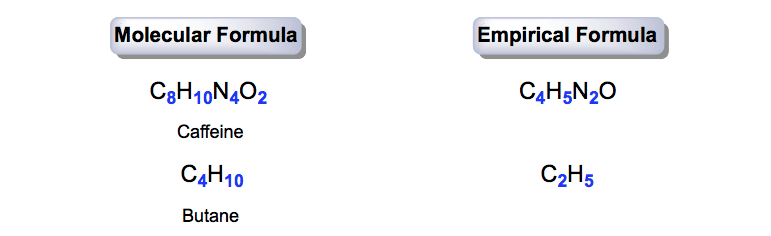Caffeine & Butane (Molecular Formulas & Empirical Formulas)

The same method of converting the molecular formulas of caffeine and butane can be applied to determine their empirical formulas.

Determining the Molecular Formula

To determine the molecular formula of a chemical compound we must first determine its empirical formula.

For example, if we are asked “A 3.927 sample of a compound was found to contain 1.536 g carbon, 0.345 g hydrogen, and the rest as oxygen. If the molecular mass of the compound is 184.18 g/mol, what is the molecular formula?” These are the steps that would need to be followed:

STEP 1: Find the mass (in grams of oxygen) by subtracting out the masses of carbon and hydrogen from the total mass of the compound.

3.927 g sample – (1.536 g C + 0.345 g H) = 2.046 g O

STEP 2: Convert the grams of each element into moles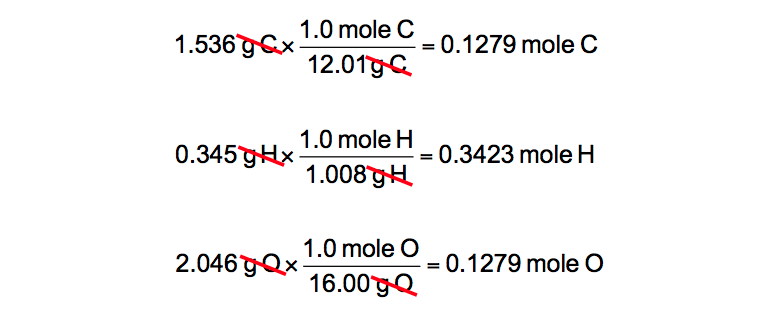Mass Conversions (Grams C, H, O to moles)

To avoid potential rounding errors, make sure you keep at least 3 decimal places for your answers in moles.

STEP 3: Divide all the moles you found in Step 2 by the smallest mole value you found to determine the empirical ratios.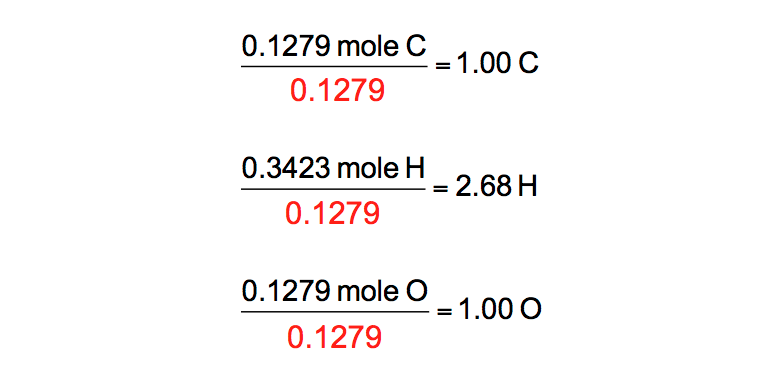Dividing by the smallest moles

STEP 4: All the empirical ratios obtained from Step 3 must be whole numbers. You can only round an empirical ratio value if it’s X.1 or X.9. For example, if you obtained 2.9 hydrogens you could round up to 3.0 hydrogens.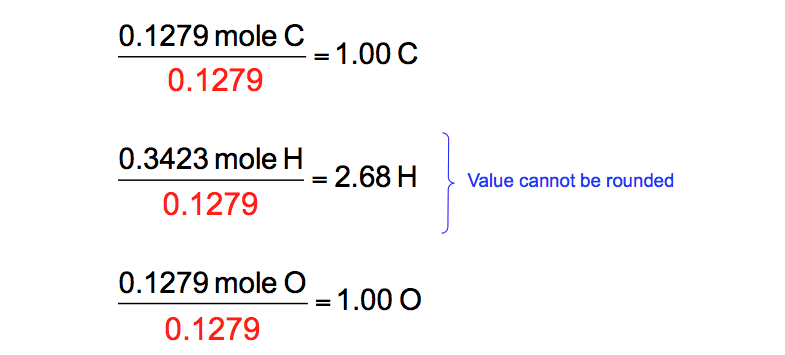Empirical Ratios & Rounding

Multiply all the empirical ratios by the same value in order to obtain whole numbers.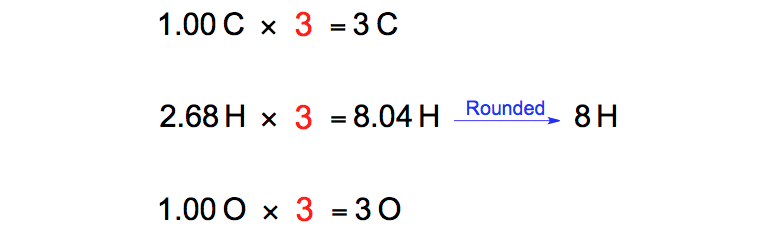Empirical Ratios, Multiplication & Whole Numbers

STEP 5: Use your new empirical ratios, which are now all whole numbers, to determine the empirical formula.Empirical Ratios & the Empirical Formula (C3H8O3)

STEP 6: We must determine an “n” value by using the following equation:Molecular Mass divided by Empirical Mass

The molecular mass or molar mass of the compound will be given within the question, but the empirical mass will be calculated from the empirical formula.Calculating molecular mass (C3H8O3)

Now that you have your “n” value you can multiply the empirical formula by it to isolate your molecular formula.Determining Molecular Formula (C3H8O3)

Empirical and molecular formulas help to determine the structural formulas of compounds. In order to accomplish the calculations needed it will important to understand the nomenclature of these compounds and the mass conversions involved.Jules Bruno

Jules felt a void in his life after his English degree from Duke, so he started tutoring in 2007 and got a B.S. in Chemistry from FIU. He’s exceptionally skilled at making concepts dead simple and helping students in covalent bonds of knowledge.